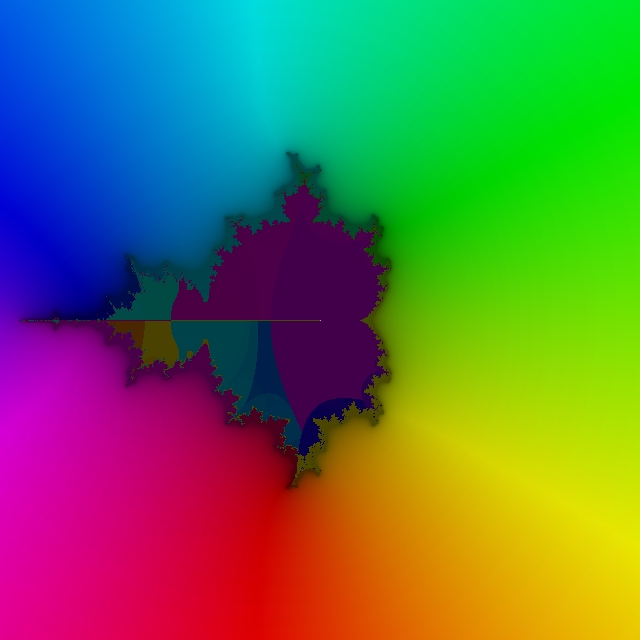[Up/Previous]

# The Mandelbrot Function

• shows the argument, or phase angle, of complex numbers in increments of 5 degrees instead of 30;
• shows the first two significant digits of the magnitude rather than the first significant digit; and
• which also shows an overlaid pattern, having a "checkerboard" appearance, from which the real and imaginary parts of the value of the function can be seen in addition to magnitude and argument.

This has required some effort, and has led to a color scheme which, admittedly, is much less clear than the one used on the previous page.

Let us illustrate the color scheme to be used here with a simple example, the identity function:Now, here's another image, this one of Gamma(z-1):Finally, this is what the Mandelbrot function looks like when drawn under this new color scheme:Note that it is probably identically zero along the portion of the real line for which the table simply gives small values.

Here is a diagram of the root of this function, using a color scheme that involves continuous colors, so as to see if my "Mandelbrot function" helps to address one difficulty with the Douady-Hubbard mapping, that it had not been possible to make it continuous:This problem is discussed on this page, with images, and that this page showed that a continuous color mapping was needed to indicate how much difficulty one has with the issue was what inspired me to check my algorithm in that manner.

It was clear enought that for points both outside the unit disk and outside the Mandelbrot Set my function should not have any problems with going to the wrong sheet of the function.

This is because the constant value added after each squaring would be smaller than the previous iteration result squared, so c would never correspond to a vector crossing the origin.

I start with c, and store the argument of c. Then, for each iteration, not only do I square z, but I also double my copy of the argument. Then, when I add c to z squared, I compare the arguments directly calculated for z squared and z squared plus c, and from that I obtain an angle to add to the argument, which I restrict to being from -180 to 180 degrees.

At the end, I repeatedly take the square root of z, but I only use the magnitude from that result, dividing the argument I kept track of explicitly by 2 to the power of the number of iterations instead.

It may well be for finer detail (which I would need to explore using a software floating-point format with a large exponent) there are still discontinuities, but it appears what I have calculated represents some progress.

[Up/Previous]